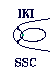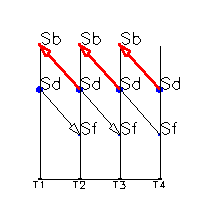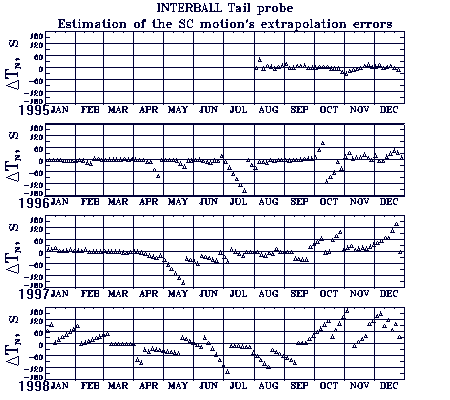### The errors estimation of the INTERBALL Tail probe motion extrapolation outside the measuring time interval

Collection of the orbital elements | Format of the orbital elements |

 The errors of the spacecraft motion extrapolation outside the measuring time interval were estimated by using the comparison of SC positions calclulated by the forward integration (extrapolation method) and backward integration (interpolation methods). The scheme of these calculations is shown on the picture by red and black vectors. Sd(i) are the SC state vectors for epoch T(i), determined on the base of the measuring time interval T(i-1), T(i), Sb(i) - the SC state vectors calculated by using backward integration from T(i+1) to T(i), with initial data Sd(i+1), Sf(i) - the SC state vectors calculated by extrapolation metod (forward integration) from T(i-1) to T(i) with initial data Sd(i-1).These calculations were fulfilled at IKI for both of the INTEBALL SC from now up to the launch.The time difference D Tn between two ascending nodes' passages calculated separately by interpolation and extrapolation methods is shown on the picture as a function of the time for each year separately from launch up to the end of the 1998. The range of the D Tn values (sec) is (-180, 180). The character of these time shift of the ascending nodes (D Tn) corresponds to the orbiting period errors, which arise during SC motion extrapolation outside the measuring intervals. The statistical estimations of these errors give the the mean square error of the orbiting period 13.6 s. In 15 days it leads to the mean square errors of 550 km in the SC position near the perigee region, and of 80 km near the apogee. These errors are the result of the non-determined character of the orbit perturbation (caused by the attitude control system) outside of the measuring intervals.
See as well the analogues estimations for the Auroral probe.